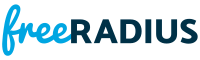snprintf.c File Reference
#include "snprintf.h"Include dependency graph for snprintf.c:

Go to the source code of this file.

Macros

#define PRECISION   1.e-6

Functions

PRIVATE void conv_flag (char *s, struct DATA *p)

PRIVATE void decimal (struct DATA *p, double d)

PRIVATE void exponent (struct DATA *p, double d)

PRIVATE void floating (struct DATA *p, double d)

PRIVATE void hexa (struct DATA *p, double d)

PRIVATE double integral (double real, double *ip)

PRIVATE int log_10 (double r)

PRIVATE char * numtoa (double number, int base, int precision, char **fract)

PRIVATE void octal (struct DATA *p, double d)

PRIVATE double pow_10 (int n)

PUBLIC int snprintf (char *string, size_t length, char *format, va_alist)

PRIVATE void strings (struct DATA *p, char *tmp)

PUBLIC int vsnprintf (char *string, size_t length, char *format, va_list args)

Macro Definition Documentation

 #define PRECISION   1.e-6

Definition at line 156 of file snprintf.c.

Function Documentation

 PRIVATE void conv_flag ( char * s, struct DATA * p )

Definition at line 451 of file snprintf.c.Here is the caller graph for this function:

 PRIVATE void decimal ( struct DATA * p, double d )

Definition at line 254 of file snprintf.c.Here is the caller graph for this function:

 PRIVATE void exponent ( struct DATA * p, double d )

Definition at line 390 of file snprintf.c.Here is the call graph for this function:Here is the caller graph for this function:

 PRIVATE void floating ( struct DATA * p, double d )

Definition at line 351 of file snprintf.c.Here is the caller graph for this function:

 PRIVATE void hexa ( struct DATA * p, double d )

Definition at line 302 of file snprintf.c.Here is the caller graph for this function:

 PRIVATE double integral ( double real, double * ip )

Definition at line 119 of file snprintf.c.Here is the call graph for this function:Here is the caller graph for this function:

 PRIVATE int log_10 ( double r )

Definition at line 91 of file snprintf.c.Here is the caller graph for this function:

 PRIVATE char* numtoa ( double number, int base, int precision, char ** fract )

Definition at line 167 of file snprintf.c.Here is the call graph for this function:

 PRIVATE void octal ( struct DATA * p, double d )

Definition at line 278 of file snprintf.c.Here is the caller graph for this function:

 PRIVATE double pow_10 ( int n )

Definition at line 65 of file snprintf.c.Here is the caller graph for this function:

 PUBLIC int snprintf ( char * string, size_t length, char * format, va_alist )

Definition at line 686 of file snprintf.c.Here is the call graph for this function:Here is the caller graph for this function:

 PRIVATE void strings ( struct DATA * p, char * tmp )

Definition at line 327 of file snprintf.c.Here is the caller graph for this function:

 PUBLIC int vsnprintf ( char * string, size_t length, char * format, va_list args )

Definition at line 503 of file snprintf.c.Here is the call graph for this function:Here is the caller graph for this function: